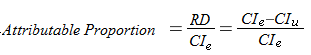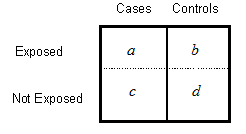# Attributable Risk / Attributable Proportion: Definition

Share on

RCTs >

## What is Attributable Risk?

Attributable Risk(AR) (sometimes called Attributable Proportion or Attributable Fraction) is a measure of the prevalence of a condition or disease. Given a group of people exposed to a risk, it’s the fraction who develop a disease or condition. Put another way, AR is the cases that would be eliminated if the exposure were also eliminated.

Often, attributable risk is given as a percentage (called the attributable risk percent or AR%). For example, lung cancer has many causes, including smoking cigarettes and exposure to indoor radon. One study showed that the AR% for cigarette smoking and lung cancer was 85%. That means 85% of lung cancers are caused by cigarette smoking. Another study showed that the AR% for radon gas exposure and lung cancer is between 3% and 20%, depending on factors like sex and smoking status.

## Formulas

The most common formula is:Where:

• RD = Risk Difference (also called Absolute Risk Reduction)
• CIe = Cumulative Incidence in Exposed group
• CIu = Cumulative Incidence in Unexposed group

## Alternate Formula

If you don’t know the cumulative incidence rates, but do know the risk or rate ratio, you can use this alternate formula:

• RR = Relative Risk

## Formula for Contingency TablesIf you have a contingency table like the one above (note the placement of the exposed/unexposed cases and controls), you can calculate the AR with the following formula:

AR = [a/(a+b)] – [c/(c+d)]

## Population Attributable Risk

The population attributable risk (PAR) (also called the population attributable fraction) is similar, except instead of a group of people (a sample) it applies to the entire population (e.g. “everyone in the United States”). The formula is:

PAR = Pe (RRe-1) / [1 + Pe (RRe-1)]

Where:

• Pe = Proportion of exposed people,
• RRe = Relative risk because of the exposure.

Like AR, PAR can also be expressed as a percentage, called population attributable risk percent (PAR%).

## Population Prevented Fraction

If the fraction is actually preventative (e.g. lives will be saved, or conditions will be prevented), then it’s called a population prevented fraction (PF) or preventive fraction. The formula is:

Pe(1-RR)

Although similar to the AR, the PF is more commonly used to measure the impact of public health interventions, where the AR is more often used in epidemiologic studies as a measure of disease prevalence.

## Attributable Number

The attributable number is a count of how many cases are attributable to a specific exposure. The formula is:

AN = Ne(Ie = Iu)

Where:

• Ne = number of people exposed.
• Ie = number of cases among exposed persons.
• Iu = number of cases among non-exposed persons.

References:
Chyou P et al. “A prospective study of the AR of cancer due to cigarette smoking.” Am J Public Health. 1992 January; 82(1): 37–40.
Kim S et al. “AR of lung cancer deaths due to indoor radon exposure”. Ann Occup Environ Med. 2016; 28: 8.
Last JM, ed., et al. A Dictionary of Epidemiology. 4th ed. New York: Oxford University Press; 2000.
Rockhill B et al. “Use and misuse of population attributable fractions”. Am J Public Health 1998;38:15-19.

CITE THIS AS:
Stephanie Glen. "Attributable Risk / Attributable Proportion: Definition" From StatisticsHowTo.com: Elementary Statistics for the rest of us! https://www.statisticshowto.com/attributable-risk/
---------------------------------------------------------------------------Need help with a homework or test question? With Chegg Study, you can get step-by-step solutions to your questions from an expert in the field. Your first 30 minutes with a Chegg tutor is free!